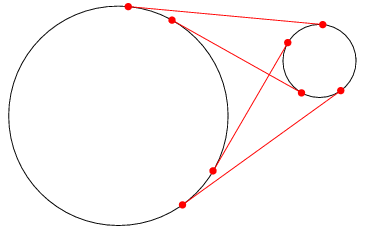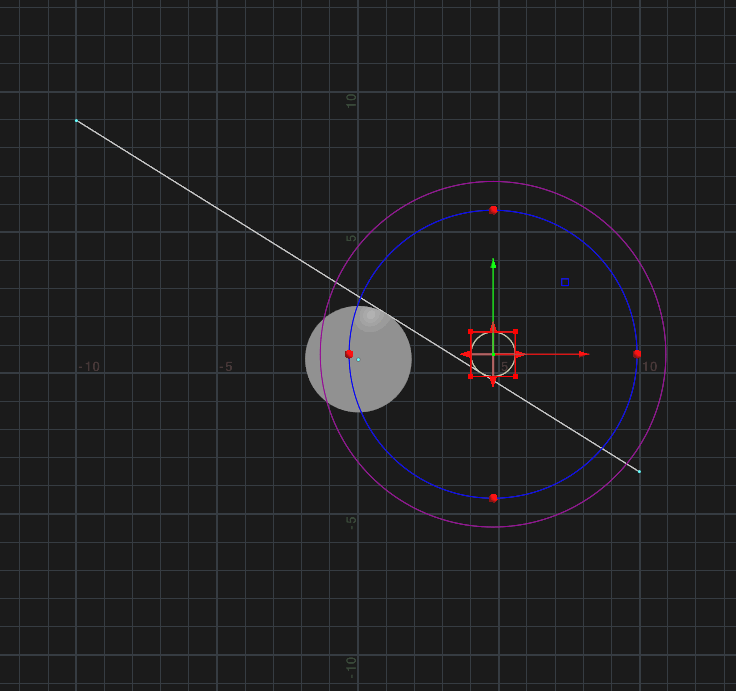# Circle tagents

## Recommended Posts

Goal:Looking into tangent lines between two circles and trying to convert this function into vex.

The code is originally in Java but looks like it should be easily converted to vex but I'm getting errors with my attempt below.

```function float[] common_tangent_line(x1, y1, r1, x2, y2, r2) {
// Compute the common tangent line of two circles: (x1, y1) - r1 and (x2, y2) - r2
// Return in the form of line equation: ax + by + c == 0
delta1 = (x1 - x2) * (x1 - x2) + (y1 - y2) * (y1 - y2) - (r1 + r2) * (r1 + r2);
delta2 = (x1 - x2) * (x1 - x2) + (y1 - y2) * (y1 - y2) - (r1 - r2) * (r1 - r2);
p1 = r1 * (x1 * x2 + y1 * y2 - x2 * x2 - y2 * y2);
p2 = r2 * (x1 * x1 + y1 * y1 - x1 * x2 - y1 * y2);
q = x1 * y2 - x2 * y1;
results = [];
if(delta1 >= 0) {
l11 = {
a: (x2 - x1) * (r1 + r2) + (y1 - y2) * Math.sqrt(delta1),
b: (y2 - y1) * (r1 + r2) + (x2 - x1) * Math.sqrt(delta1),
c: p1 + p2 + q * Math.sqrt(delta1)
};
l12 = {
a: (x2 - x1) * (r1 + r2) - (y1 - y2) * Math.sqrt(delta1),
b: (y2 - y1) * (r1 + r2) - (x2 - x1) * Math.sqrt(delta1),
c: p1 + p2 - q * Math.sqrt(delta1)
};
results.push(l11);
results.push(l12);
}
if(delta2 >= 0) {
l21 = {
a: (x2 - x1) * (r1 - r2) + (y1 - y2) * Math.sqrt(delta2),
b: (y2 - y1) * (r1 - r2) + (x2 - x1) * Math.sqrt(delta2),
c: p1 - p2 + q * Math.sqrt(delta2)
};
l22 = {
a: (x2 - x1) * (r1 - r2) - (y1 - y2) * Math.sqrt(delta2),
b: (y2 - y1) * (r1 - r2) - (x2 - x1) * Math.sqrt(delta2),
c: p1 - p2 - q * Math.sqrt(delta2)
};
results.push(l21);
results.push(l22);
}
return results;
}```

Edited by sessionbeer
##### Share on other sites

Hi,

You also have to change the function calls like:

```Math.sqrt(delta1)

results.push(l21)```

to:

```sqrt(delta1)

push(results, l21)```

##### Share on other sites

Thanks! I'm getting an error with the first line:

```function float[] common_tangent_line(x1, y1, r1, x2, y2, r2) {
....
}
```

Error: Syntax error, unexpected ',', expecting identifies (1,40).

##### Share on other sites

You have to specify the type for the arguments:

```function float[] common_tangent_line(float x1, y1, r1, x2, y2, r2) {

}```

##### Share on other sites

Great, thank you. Last one:
How do I correct the error for a: ?

```if(delta1 >= 0) {
l11 = {
a: = (x2 - x1) * (r1 + r2) + (y1 - y2) * sqrt(delta1),
b: (y2 - y1) * (r1 + r2) + (x2 - x1) * sqrt(delta1),
c: p1 + p2 + q * sqrt(delta1)
};```

##### Share on other sites

11 hours ago, sessionbeer said:

Great, thank you. Last one:
How do I correct the error for a: ?

```
if(delta1 >= 0) {
l11 = {
a: = (x2 - x1) * (r1 + r2) + (y1 - y2) * sqrt(delta1),
b: (y2 - y1) * (r1 + r2) + (x2 - x1) * sqrt(delta1),
c: p1 + p2 + q * sqrt(delta1)
};```

Looks like homework wasn't done first##### Share on other sites

Really hard to do the right homework when you don't know the right questions to askEdited by sessionbeer
##### Share on other sites

Getting no more errors but seems like the results are incorrect. I've made a @common attribute to hold the results which I'm expecting to be (a,b,c) but instead, I'm getting a lot more float values. Attached a hip file too to show all the errors in my ways.

```function float[] common_tangent_line(float x1, y1, r1, x2, y2, r2) {
// Compute the common tangent line of two circles: (x1, y1) - r1 and (x2, y2) - r2
// Return in the form of line equation: ax + by + c == 0
float delta1 = (x1 - x2) * (x1 - x2) + (y1 - y2) * (y1 - y2) - (r1 + r2) * (r1 + r2);
float delta2 = (x1 - x2) * (x1 - x2) + (y1 - y2) * (y1 - y2) - (r1 - r2) * (r1 - r2);
float p1 = r1 * (x1 * x2 + y1 * y2 - x2 * x2 - y2 * y2);
float p2 = r2 * (x1 * x1 + y1 * y1 - x1 * x2 - y1 * y2);
float q = x1 * y2 - x2 * y1;
float results[];

if(delta1 >= 0) {
float a = (x2 - x1) * (r1 + r2) + (y1 - y2) * sqrt(delta1);
float b = (y2 - y1) * (r1 + r2) + (x2 - x1) * sqrt(delta1);
float c = p1 + p2 + q * sqrt(delta1);
float   l11[];
l11 = array(a,b,c);

float a2 = (x2 - x1) * (r1 + r2) - (y1 - y2) * sqrt(delta1);
float b2 = (y2 - y1) * (r1 + r2) - (x2 - x1) * sqrt(delta1);
float c2 = p1 + p2 - q * sqrt(delta1);
float   l12[];
l12 = array(a,b,c);
push(results, l11);
push(results, l12);
};
if(delta2 >= 0) {
float a = (x2 - x1) * (r1 - r2) + (y1 - y2) * sqrt(delta2);
float b = (y2 - y1) * (r1 - r2) + (x2 - x1) * sqrt(delta2);
float c = p1 - p2 + q * sqrt(delta2);
float   l21[];
l21 = array(a,b,c);

float a2 = (x2 - x1) * (r1 - r2) - (y1 - y2) * sqrt(delta2);
float b2 = (y2 - y1) * (r1 - r2) - (x2 - x1) * sqrt(delta2);
float c2 = p1 - p2 - q * sqrt(delta2);
float   l22[];
l22 = array(a,b,c);
push(results, l21);
push(results, l22);
}
return results;
}

vector point1 = @P;;
vector point2 = (0,"P",1);

f[]@common =  common_tangent_line(point1.x, point1.y, 1.5, point2.x, point2.y, 1);```

Edited by sessionbeer
##### Share on other sites

10 hours ago, sessionbeer said:

Getting no more errors but seems like the results are incorrect. I've made a @common attribute to hold the results which I'm expecting to be (a,b,c) but instead, I'm getting a lot more float values. Attached a hip file too to show all the errors in my ways.

```
function float[] common_tangent_line(float x1, y1, r1, x2, y2, r2) {
// Compute the common tangent line of two circles: (x1, y1) - r1 and (x2, y2) - r2
// Return in the form of line equation: ax + by + c == 0
float delta1 = (x1 - x2) * (x1 - x2) + (y1 - y2) * (y1 - y2) - (r1 + r2) * (r1 + r2);
float delta2 = (x1 - x2) * (x1 - x2) + (y1 - y2) * (y1 - y2) - (r1 - r2) * (r1 - r2);
float p1 = r1 * (x1 * x2 + y1 * y2 - x2 * x2 - y2 * y2);
float p2 = r2 * (x1 * x1 + y1 * y1 - x1 * x2 - y1 * y2);
float q = x1 * y2 - x2 * y1;
float results[];

if(delta1 >= 0) {
float a = (x2 - x1) * (r1 + r2) + (y1 - y2) * sqrt(delta1);
float b = (y2 - y1) * (r1 + r2) + (x2 - x1) * sqrt(delta1);
float c = p1 + p2 + q * sqrt(delta1);
float   l11[];
l11 = array(a,b,c);

float a2 = (x2 - x1) * (r1 + r2) - (y1 - y2) * sqrt(delta1);
float b2 = (y2 - y1) * (r1 + r2) - (x2 - x1) * sqrt(delta1);
float c2 = p1 + p2 - q * sqrt(delta1);
float   l12[];
l12 = array(a,b,c);
push(results, l11);
push(results, l12);
};
if(delta2 >= 0) {
float a = (x2 - x1) * (r1 - r2) + (y1 - y2) * sqrt(delta2);
float b = (y2 - y1) * (r1 - r2) + (x2 - x1) * sqrt(delta2);
float c = p1 - p2 + q * sqrt(delta2);
float   l21[];
l21 = array(a,b,c);

float a2 = (x2 - x1) * (r1 - r2) - (y1 - y2) * sqrt(delta2);
float b2 = (y2 - y1) * (r1 - r2) - (x2 - x1) * sqrt(delta2);
float c2 = p1 - p2 - q * sqrt(delta2);
float   l22[];
l22 = array(a,b,c);
push(results, l21);
push(results, l22);
}
return results;
}

vector point1 = @P;;
vector point2 = (0,"P",1);

f[]@common =  common_tangent_line(point1.x, point1.y, 1.5, point2.x, point2.y, 1);```

Well done. Check hip, I have added the rest code from the article above. Hope it will help to finish the setup.Edited by fencer
##### Share on other sites

Thanks for all the helpful tips, I got it working finally## Join the conversation

You can post now and register later. If you have an account, sign in now to post with your account.
Note: Your post will require moderator approval before it will be visible.×   Pasted as rich text.   Paste as plain text instead

Only 75 emoji are allowed.

×   Your previous content has been restored.   Clear editor

×   You cannot paste images directly. Upload or insert images from URL.

×
×